# frest.Chirp

Swept-frequency cosine input signal

## Description

Use a `frest.Chirp` object to represent a swept-frequency cosine input signal for frequency response estimation. A swept-frequency cosine input signal, or chirp signal, excites your system at a range of frequencies, such that the input frequency changes instantaneously.

Chirp signals are useful when your system is nearly linear in the simulation range. They are also useful when you want to obtain a response quickly for a lot of frequency points. The frequency-response model that results when you use a chirp input contains only frequencies that fall within the range of the chirp.

You can use a chirp input signal for estimation at the command line, in the Model Linearizer, or with the Frequency Response Estimator block. The estimation algorithm injects the signal at the input point you specify for estimation, and measures the response at the output point. For more information, see Chirp Input Signals.

To view a plot of your input signal, type `plot(input)`. To create a `timeseries` object for your input signal, use the `generateTimeseries` command.

## Creation

### Syntax

``input = frest.Chirp(sys)``
``input = frest.Chirp(Name,Value)``

### Description

````input = frest.Chirp(sys)` creates a swept-frequency cosine input signal with properties based on the dynamics of the linear system `sys`. For instance, if you have an exact linearization of your system, you can use it to initialize the parameters.```

example

````input = frest.Chirp(Name,Value)` creates a swept-frequency cosine input signal with properties specified using one or more name-value pairs. Enclose each property name in quotes.```

### Input Arguments

expand all

Linear dynamic system, specified as a SISO `ss`, `tf`, or `zpk` object. You can specify known dynamics or obtain the linear model by linearizing a nonlinear system.

The resulting chirp signal automatically sets these options based on the linear system:

• `FreqRange` are the frequencies at which the linear system has interesting dynamics.

• `Ts` is set to avoid aliasing such that the Nyquist frequency of the signal is five times the upper end of the frequency range.

• `NumSamples` is set such that the frequency response estimation includes the lower end of the frequency range.

The remaining properties use default values.

## Properties

expand all

Signal amplitude at each frequency, specified as a positive scalar.

Signal frequency range, specified as one of the following:

• Two-element vector, for example `[w1 w2]`

• Two-element cell array, for example `{w1 w2}`

Here, `w1` is the lower bound of the frequency range, and `w2` is the upper bound.

Frequency units, specified as one of the following:

• `'rad/s'` — Radians per second

• `'Hz'` — Hertz

Changing frequency units does not impact frequency response estimation.

Sample time of the chirp signal in seconds, specified as a positive scalar. The default sample time, which avoids aliasing, is:

`$\frac{2\pi }{5*\mathrm{max}\left(FreqRange\right)}$`

Here, FreqRange is specified in rad/s.

Number of samples in the chirp signal, specified as a positive integer. The default number of samples, which ensures that the estimation includes the lower end of the frequency range, is:

`$\frac{4\pi }{Ts*\mathrm{min}\left(FreqRange\right)}$`

Here, FreqRange is specified in rad/s.

This property does not determine number of frequency points in the final estimation result. The `frestimate` function only includes frequency points with positive values. The function also discards any frequencies that fall outside the frequency range specified for the chirp.

Method for evolution of instantaneous frequency, specified as one of the following.

MethodDescription
`'linear'`

Specifies the instantaneous frequency sweep fi(t):

`${f}_{i}\left(t\right)={f}_{0}+\beta t\text{\hspace{0.17em}}\text{\hspace{0.17em}}where\text{\hspace{0.17em}}\text{\hspace{0.17em}}\beta =\left({f}_{1}-{f}_{0}\right)/{t}_{f}$`

β ensures that the signal maintains the desired frequency breakpoint f1 at final time tf.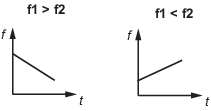`'logarithmic'`

Specifies the instantaneous frequency sweep fi(t):

`${f}_{i}\left(t\right)={f}_{0}×{\beta }^{t}\text{\hspace{0.17em}}\text{\hspace{0.17em}}where\text{\hspace{0.17em}}\text{\hspace{0.17em}}\beta ={\left(\frac{{f}_{1}}{{f}_{0}}\right)}^{\frac{1}{{t}_{f}}}$`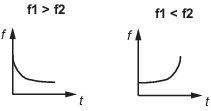`'quadratic'`

Specifies the instantaneous frequency sweep fi(t):

`${f}_{i}\left(t\right)={f}_{0}+\beta {t}^{2}\text{\hspace{0.17em}}\text{\hspace{0.17em}}where\text{\hspace{0.17em}}\text{\hspace{0.17em}}\beta =\left({f}_{1}-{f}_{0}\right)/{t}_{i}^{2}$`

Specify the shape of the quadratic using the `Shape` option.

Quadratic sweep parabola shape, specified as one of the following:

• `'concave'` — Concave quadratic sweeping shape.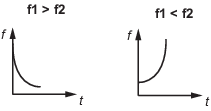• `'convex'` — Convex quadratic sweeping shape.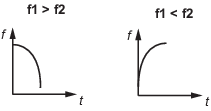This property is available on when `SweepMethod` is `'quadratic'`.

Initial phase of the Chirp signal in degrees, specified as a scalar.

## Object Functions

 `frestimate` Frequency response estimation of Simulink models `generateTimeseries` Generate time-domain data for input signal `frest.simCompare` Plot time-domain simulation of nonlinear and linear models `frest.simView` Plot frequency response model in time- and frequency-domain `getSimulationTime` Final time of simulation for frequency response estimation

## Examples

collapse all

Create a chirp input signal with frequencies ranging from 10 to 500 rad/s. Specify the amplitude and the number of samples as well.

```input = frest.Chirp('Amplitude',1e-3,... 'FreqRange',[10 500],... 'NumSamples',750)```
``` The chirp input signal: FreqRange : [10 500] (rad/s) Amplitude : 0.001 Ts : 0.00251327412287183 (sec) NumSamples : 750 InitialPhase : 270 (deg) FreqUnits (rad/s or Hz): rad/s SweepMethod(linear/ : linear quadratic/ logarithmic) ```

Plot the chirp signal.

`plot(input)`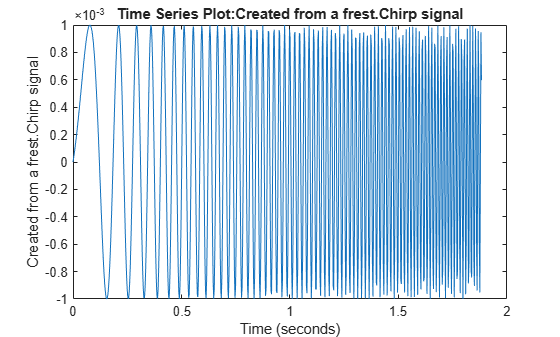Create a chirp input signal based on the dynamics of a linear system. This approach is useful when you are using frequency response estimation to validate the linearization of your model.

```model = 'watertank'; open_system(model) ```For this example, linearize the model at a steady-state operating point to obtain a state-space model you can use to initialize the chirp signal.

```io(1)=linio('watertank/PID Controller',1,'input'); io(2)=linio('watertank/Water-Tank System',1,'openoutput'); watertank_spec = operspec(model); opOpts = findopOptions('DisplayReport','off'); op = findop(model,watertank_spec,opOpts); sys = linearize(model,op,io); ```

Create the chirp signal.

```input = frest.Chirp(sys); ```

`frest.Chirp` chooses a frequency range based on the system dynamics. It also automatically initializes other parameters of the chirp signal.

```input ```
``` The chirp input signal: FreqRange : [0.001581138830107 0.1581138830107] (rad/s) Amplitude : 1e-05 Ts : 7.94767061252222 (sec) NumSamples : 1000 InitialPhase : 270 (deg) FreqUnits (rad/s or Hz): rad/s SweepMethod(linear/ : linear quadratic/ logarithmic) ```

You can change properties of the signal using dot notation. For instance, increase the signal amplitude.

```input.Amplitude = 3e-5 ```
``` The chirp input signal: FreqRange : [0.001581138830107 0.1581138830107] (rad/s) Amplitude : 3e-05 Ts : 7.94767061252222 (sec) NumSamples : 1000 InitialPhase : 270 (deg) FreqUnits (rad/s or Hz): rad/s SweepMethod(linear/ : linear quadratic/ logarithmic) ```

## Alternative Functionality

### Model Linearizer

In the Model Linearizer, to use a chirp input signal for estimation, on the Estimation tab, select Input Signal > Chirp.